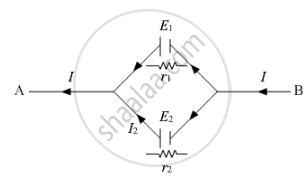# Two Cells of Emfs E1 and E2 and Internal Resistances R1 and R2 Are Connected in Parallel. Derive the Expression for the (I) Emf and (Ii) Internal Resistance of a Single Equivalent Cell Which Can Replace this Combination. - Physics

Two cells of emf E1 and E2 and internal resistances r1 and r2 are connected in parallel. Derive the expression for the (i) emf and (ii) internal resistance of a single equivalent cell which can replace this combination.

#### Solutionwhere

E1, E2 = Emf of two cells

r1, r2 = Internal resistances of cell

I1, I2 = Current due to the two cells

Terminal potential difference across the first cell is

V = E1 − I1r1

=>I_1=(E_1-V)/r_1

For the second cell, terminal potential difference will be equal to that across the first cell. So,

V = E2I2r2

⇒I_2=(E_2−V)/r_2

Let E be effective emf and r is effective internal resistance. Let I be the current flowing through the cell.

I=I1+I2

⇒I=(E1−V)/r_1+(E2−V)/r_2

⇒Ir_1r_2=E_1r_2+E_1r_1−(r_1+r_2)V

=>V=(E_1r_2+E_2r_1)/(r_1+r_2)−(Ir_1r_2)/(r_1+r_2)

Comparing the equation with V=EIr, we get

E=(E_1r_2+E_2r_1)/(r_1+r_2)

r=(r_1r_2)/(r_1+r_2)

Concept: Induced Emf and Current
Is there an error in this question or solution?